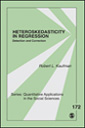Little Green Books

# Heteroskedasticity in Regression: Detection and Correction

This volume covers the commonly ignored topic of heteroskedasticity (unequal error variances) in regression analyses and provides a practical guide for how to proceed in terms of testing and correction. Emphasizing how to apply diagnostic tests and corrections for heteroskedasticity in actual data analyses, the book offers three approaches for dealing with heteroskedasticity:

variance-stabilizing transformations of the dependent variable; calculating robust standard errors, or heteroskedasticity-consistent standard errors; and; generalized least squares estimation coefficients and standard errors.

The detection and correction of heteroskedasticity is illustrated with three examples that vary in terms of sample size and the types of units analyzed (individuals, households, U.S. states). Intended as a supplementary text for graduate-level courses and a primer for quantitative researchers, the book fills the gap between the limited coverage of heteroskedasticity provided in applied regression textbooks and the more theoretical statistical treatment in advanced econometrics textbooks.

•••